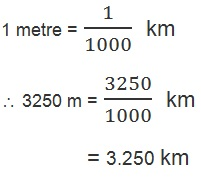Study Materials

# NCERT Solutions for Class 6th Science

Page 2 of 4

## Chapter 10. MOTION AND MEASUREMENT OF DISTANCES

### Text book Exercise:

Q1. Give two examples each, of modes of transport used on land, water and air.

Ans: Two examples of each.

Land transport: bus and train.

Water transport: boat and   ship.

Air transport: Helicopter and aeroplane.

Q2. Fill in the blanks:
(i) One metre is ______________ cm.
(ii) Five kilometre is ______________ m.
(iii)Motion of a child on a swing is ______________.
(iv) Motion of the needle of a sewing machine is ______________.
(v) Motion of wheel of a bicycle is______________.

Ans:

(i) 100

(ii)  500

(iii) periodic motion

(iv) periodic motion

(v)  rotational motion

Q3. Why can a pace or a footstep not be used as a standard unit of length?

Ans: Standarrd unit of length must have a fixed numeric value while footstep does have so.

Q4. Arrange the following lengths in their increasing magnitude:
1 metre, 1 centimetre, 1 kilometre,1 millimetre.

Ans: 1 milimetre, 1 centimetre, 1 metre, 1 kilometre

Q5. The height of a person is 1.65 m. Express it into cm and mm.

Ans:

The height of a person = 1.65 m

In centimetre,

1 metre = 100 cm

1.65 m = 1.65 x 100 cm

= 165 cm

In milimetre,

1 centimetre = 10 milimetre

1 metre = 100 centimetre

Or        = 100 x 10 milimetre

= 1000 milimetre

1.65 metre = 1.65 x 1000 milimetre

= 1650 milimetre

Q6. The distance between Radha's home and her school is 3250 m. Express this distance into km.

Ans:Q7. While measuring the length of a knitting needle, the reading of the scale at one end is 3.0 cm and at the other end is 33.1 cm. What is the length of the needle?

Ans:

Reading of scale at one end = 3.0 cm

Reading of scale at other end = 33.1 cm

Distance between two end = 33.1 - 3.0 cm

= 30.1 cm

Q8. Write the similarities and differences between the motion of a bicycle and a
ceiling fan that has been switched on.

Ans:

Similarities: the motion of a bicycle and a celing fan both have circular motion.

Differences: The bicycle moves on straight line but ceiling fan does not move so.

Q9. Why could you not use an elastic measuring tape to measure distance? What would be some of the problems you would meet in telling someone about a distance you measured with an elastic tape?

Ans:

Q10. Give two examples of periodic motion.

Ans:

(i) The motion of child on a swing.

(ii) moving here and there the branches of tree.

Page 2 of 4

Chapter Contents: